# RS Aggarwal Solutions For Class 7 Maths Exercise 1B Chapter 1 Integers

The pdf of RS Aggarwal Solutions for the Exercise 1B of Class 7 Maths Chapter 1, Integers are provided here. To cover the entire syllabus in Maths, the RS Aggarwal is an essential material as it offers a wide range of questions that test the students understanding of concepts. Our expert personnel have solved the problems step by step with neat explanations. Students who want to score good marks in Maths, then refer RS Aggarwal Solutions for Class 7.

## Download the PDF of RS Aggarwal Solutions For Class 7 Maths Chapter 1 Integers – Exercise 1B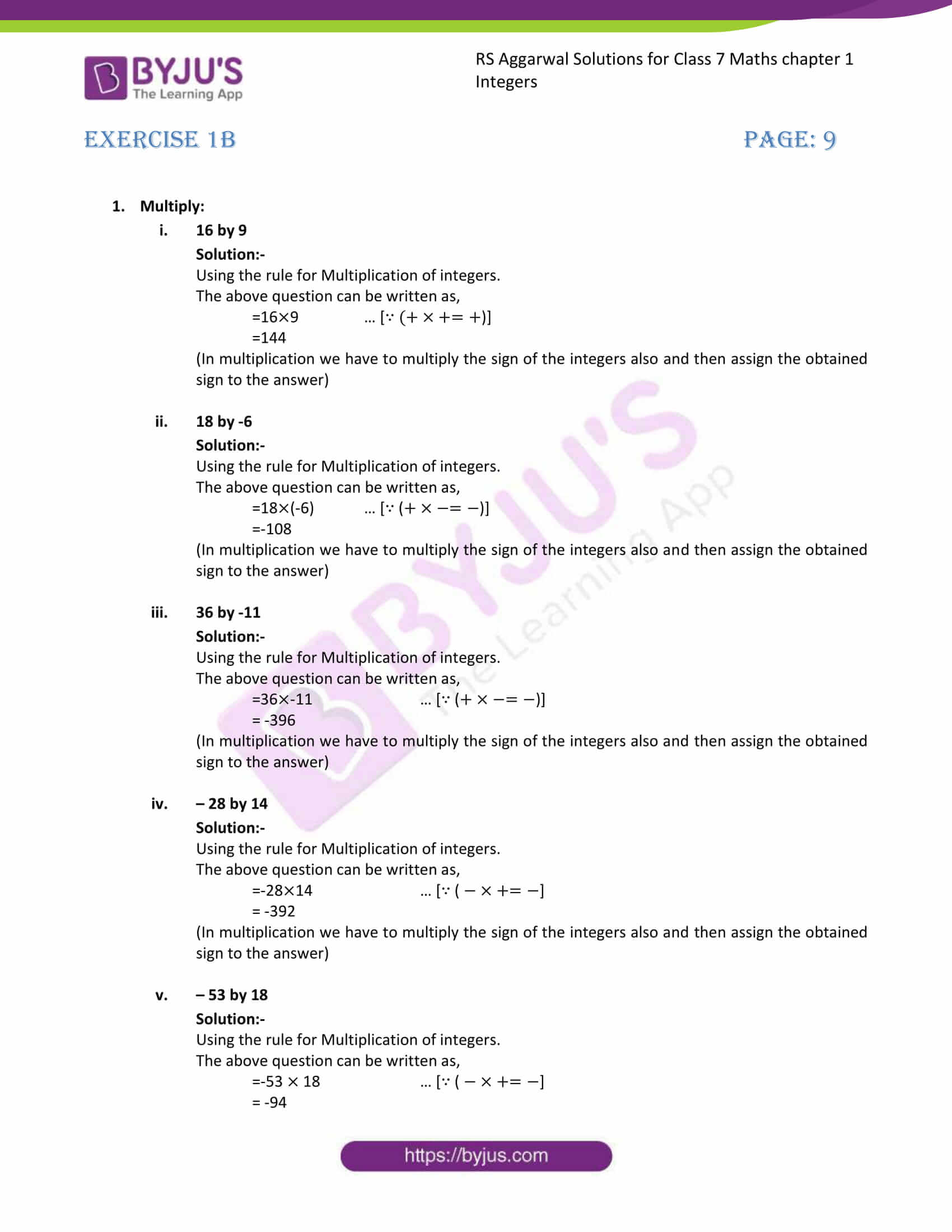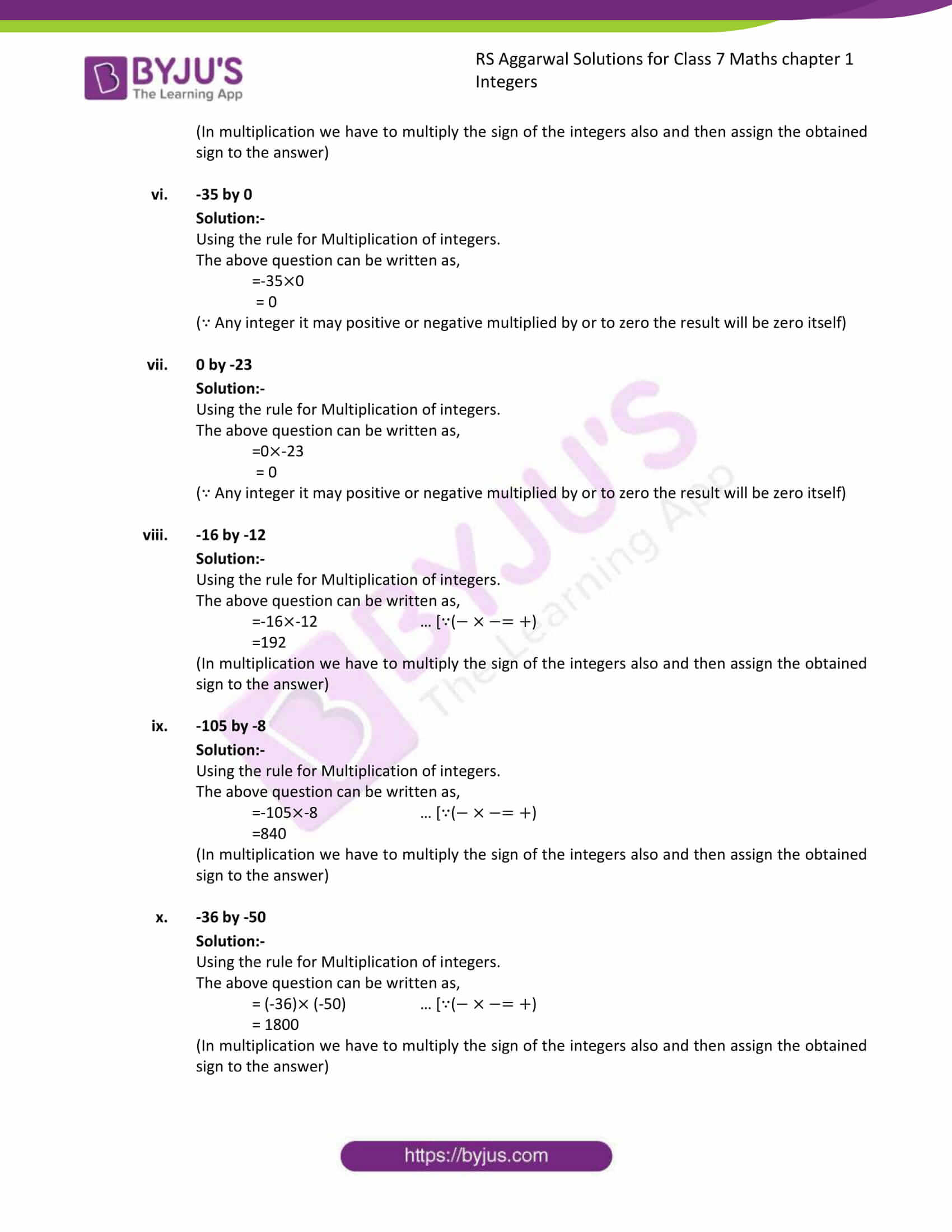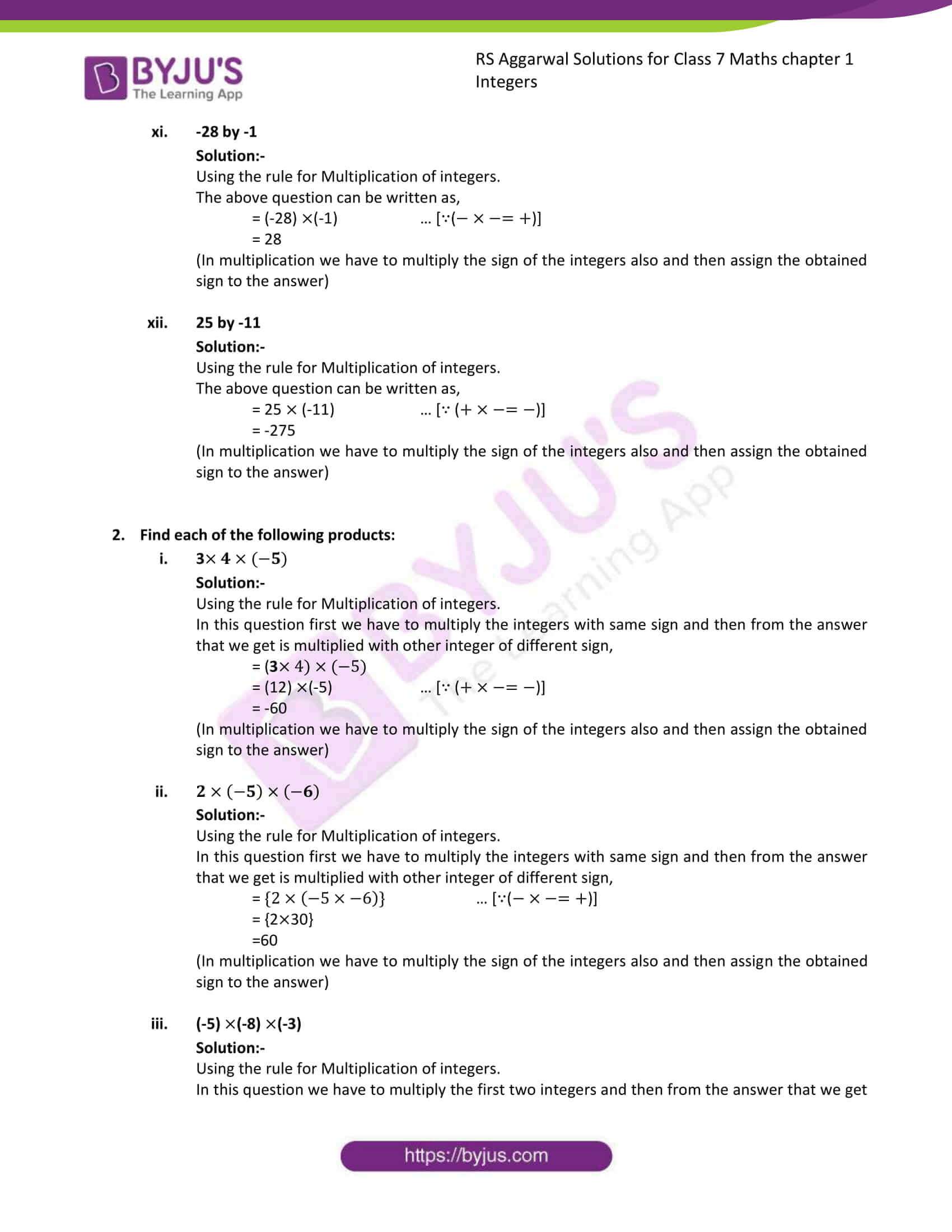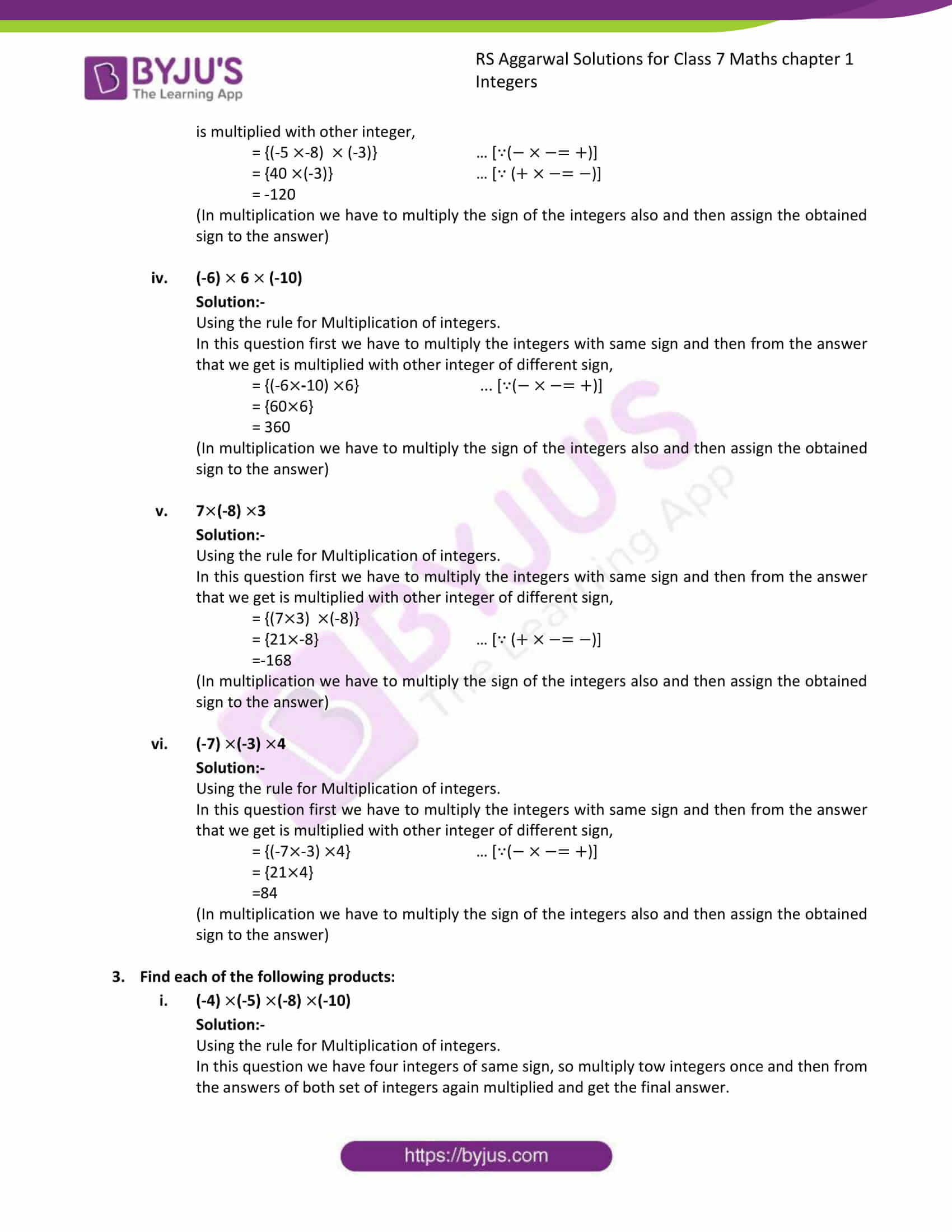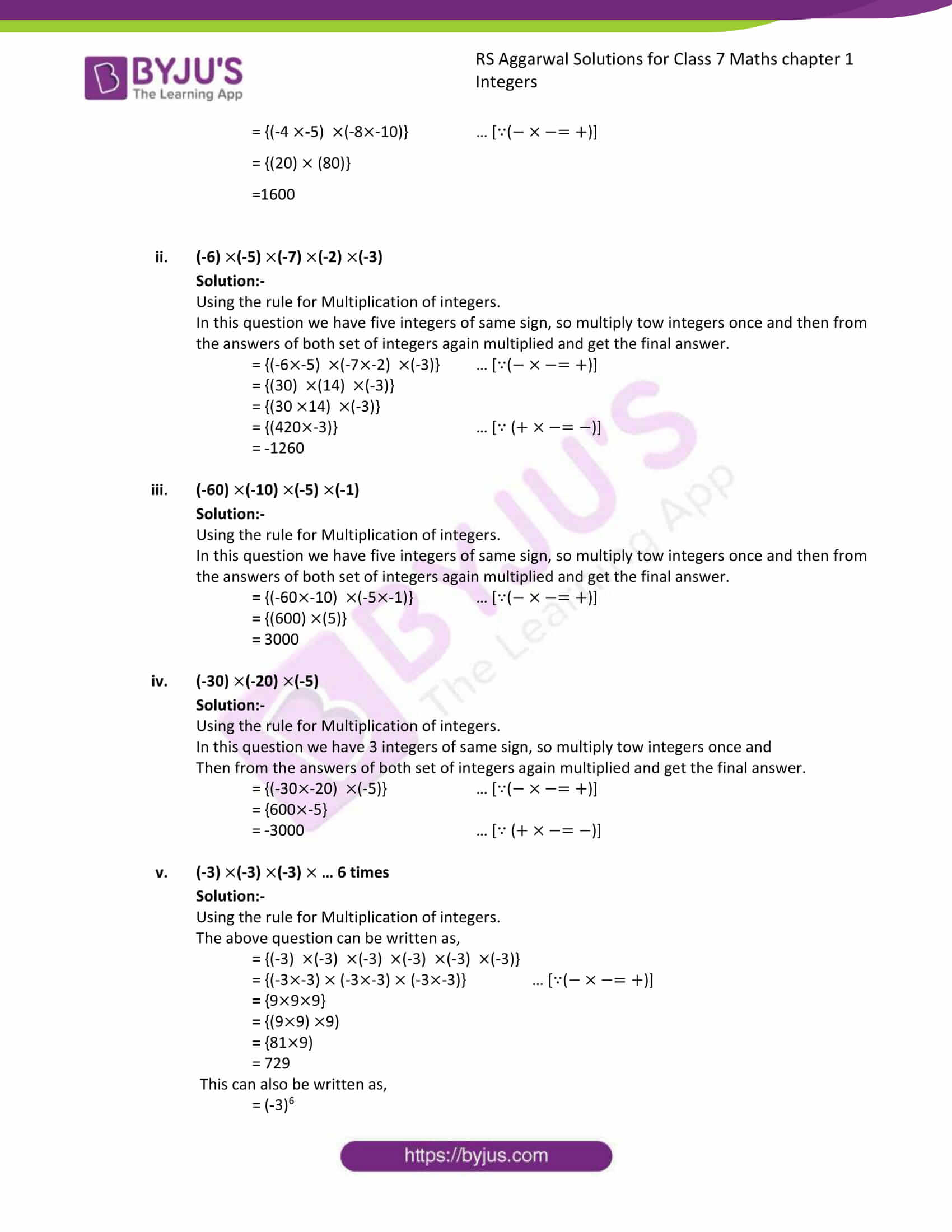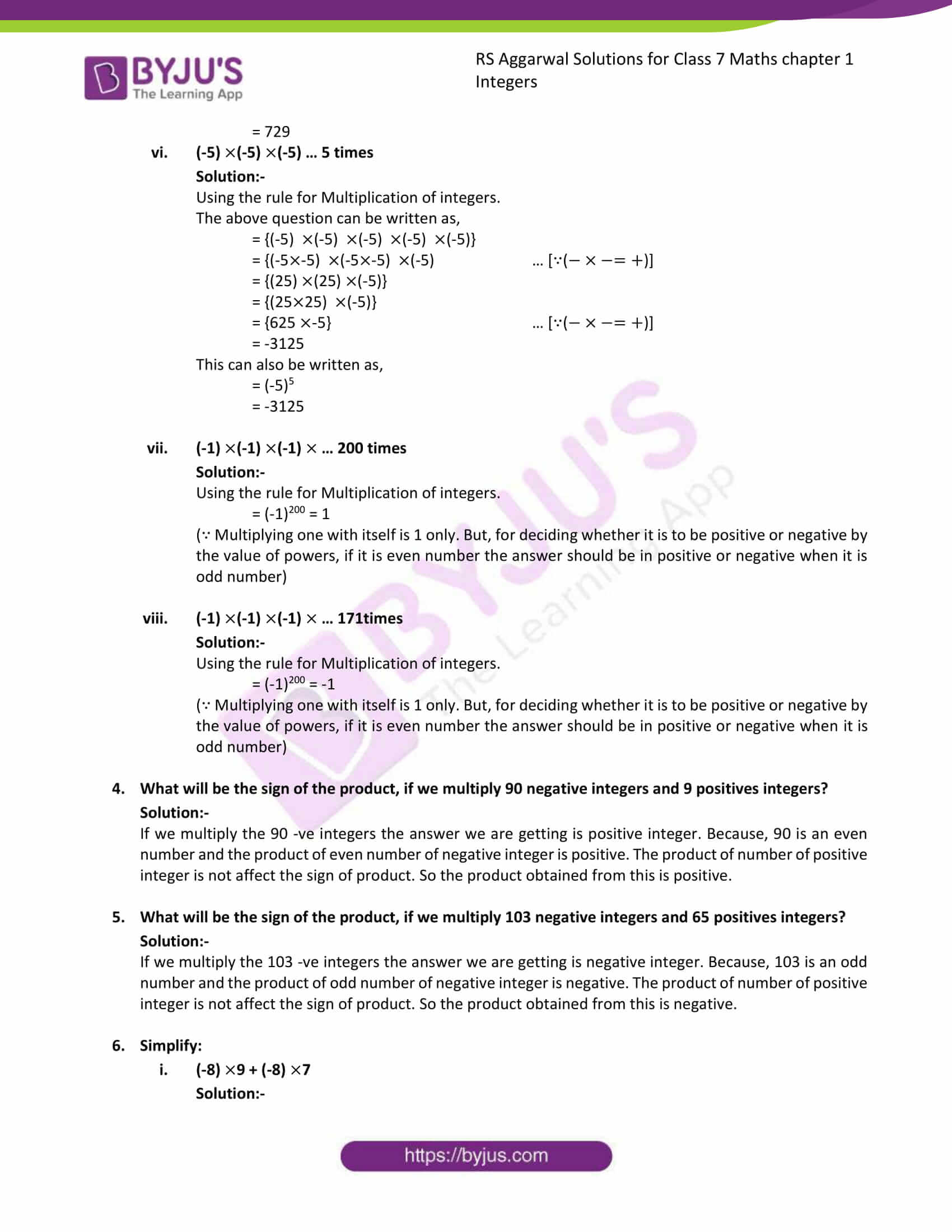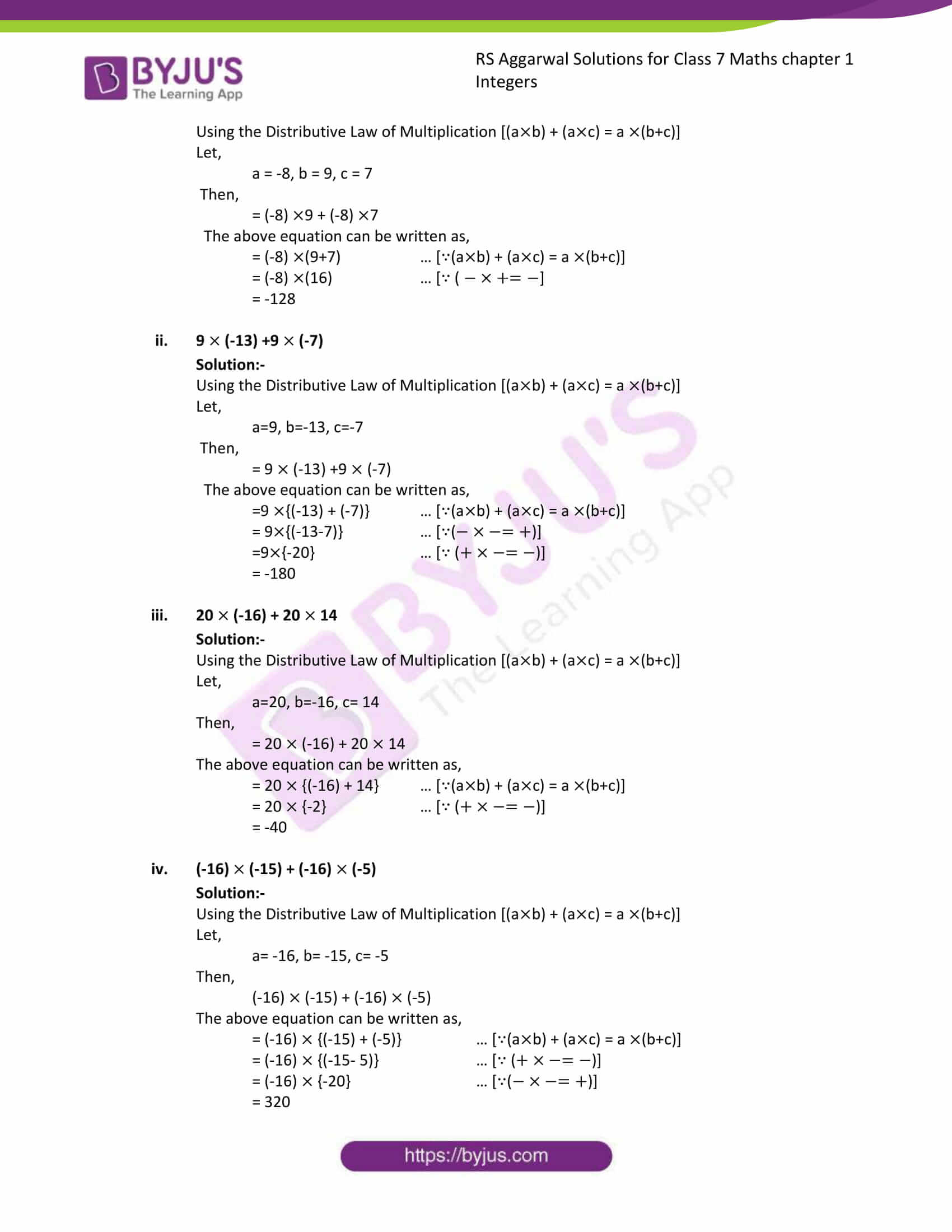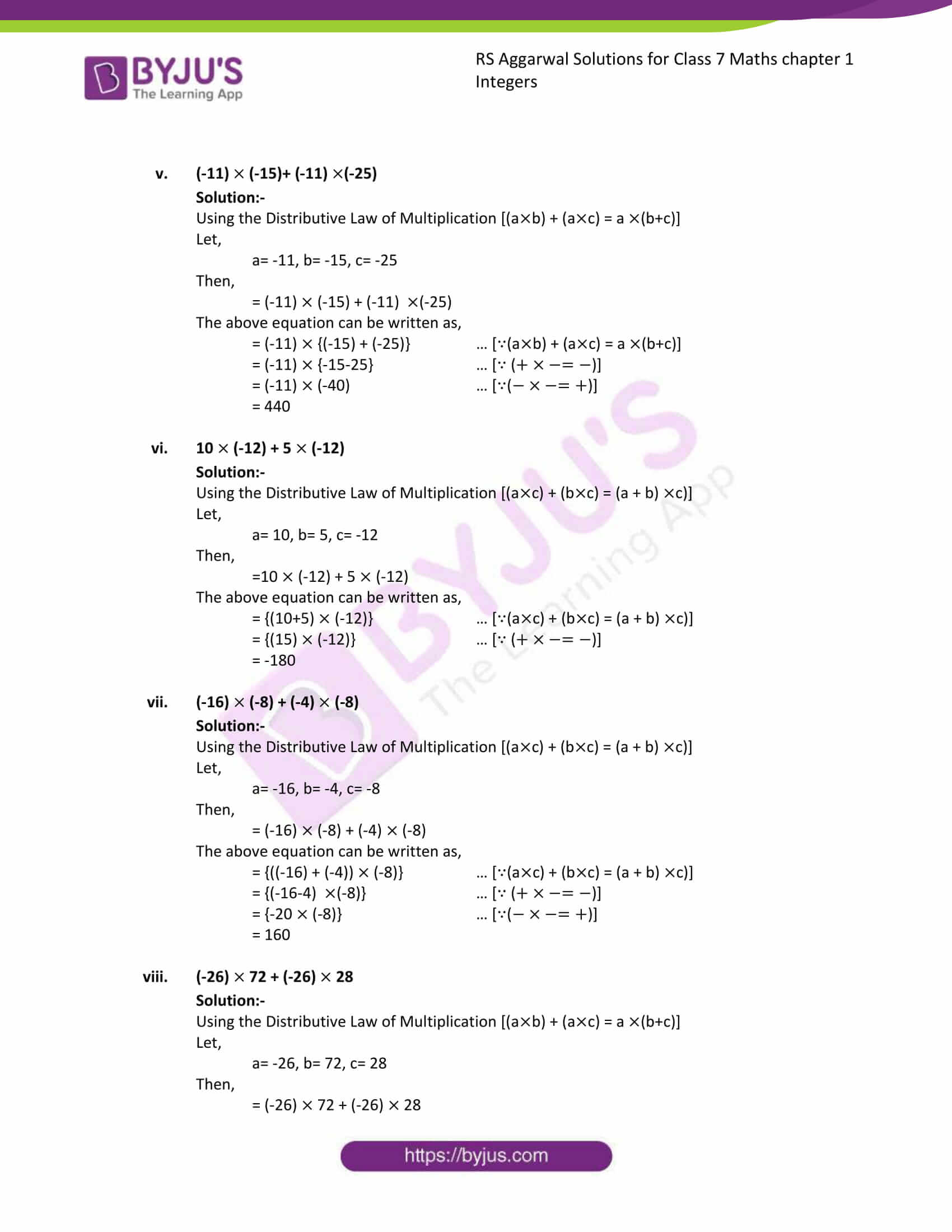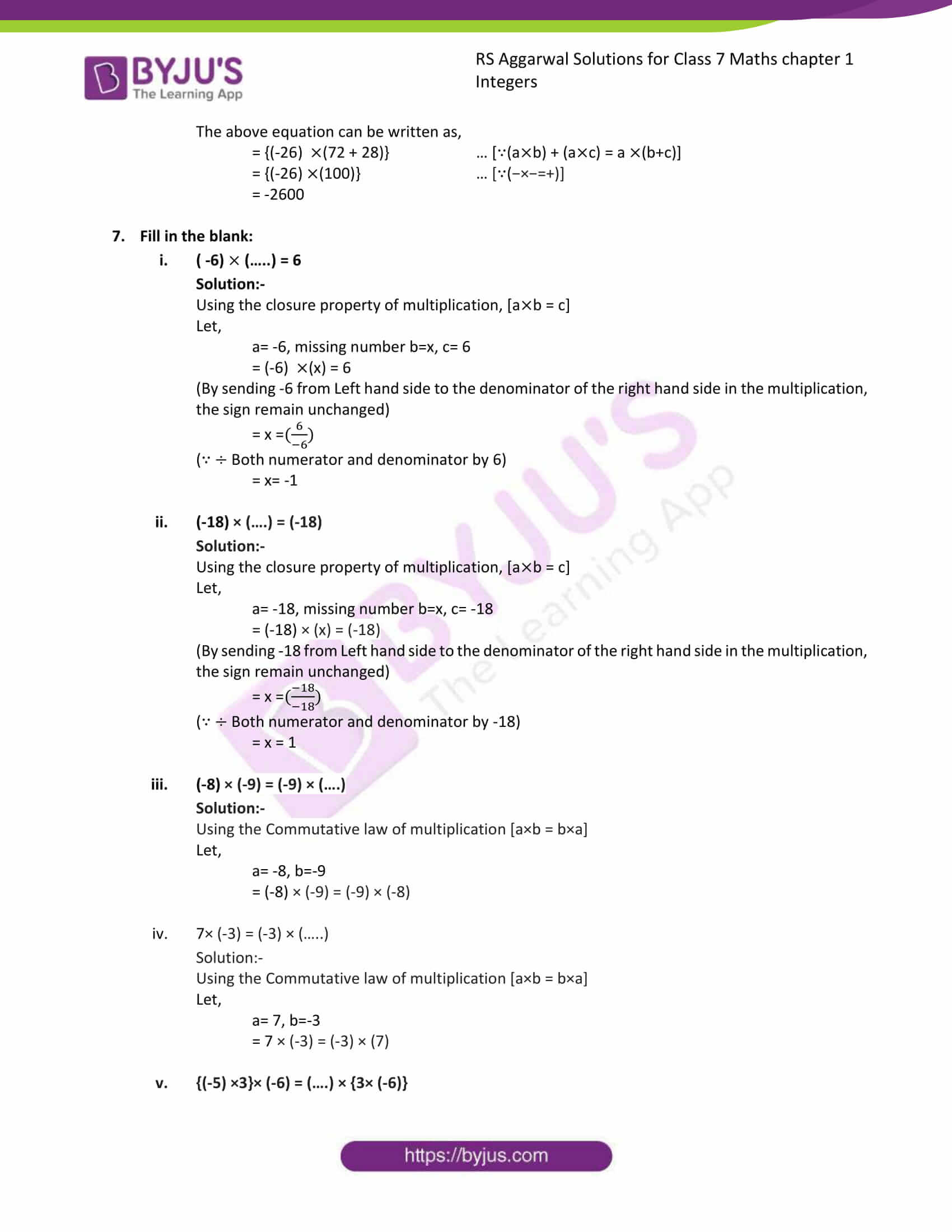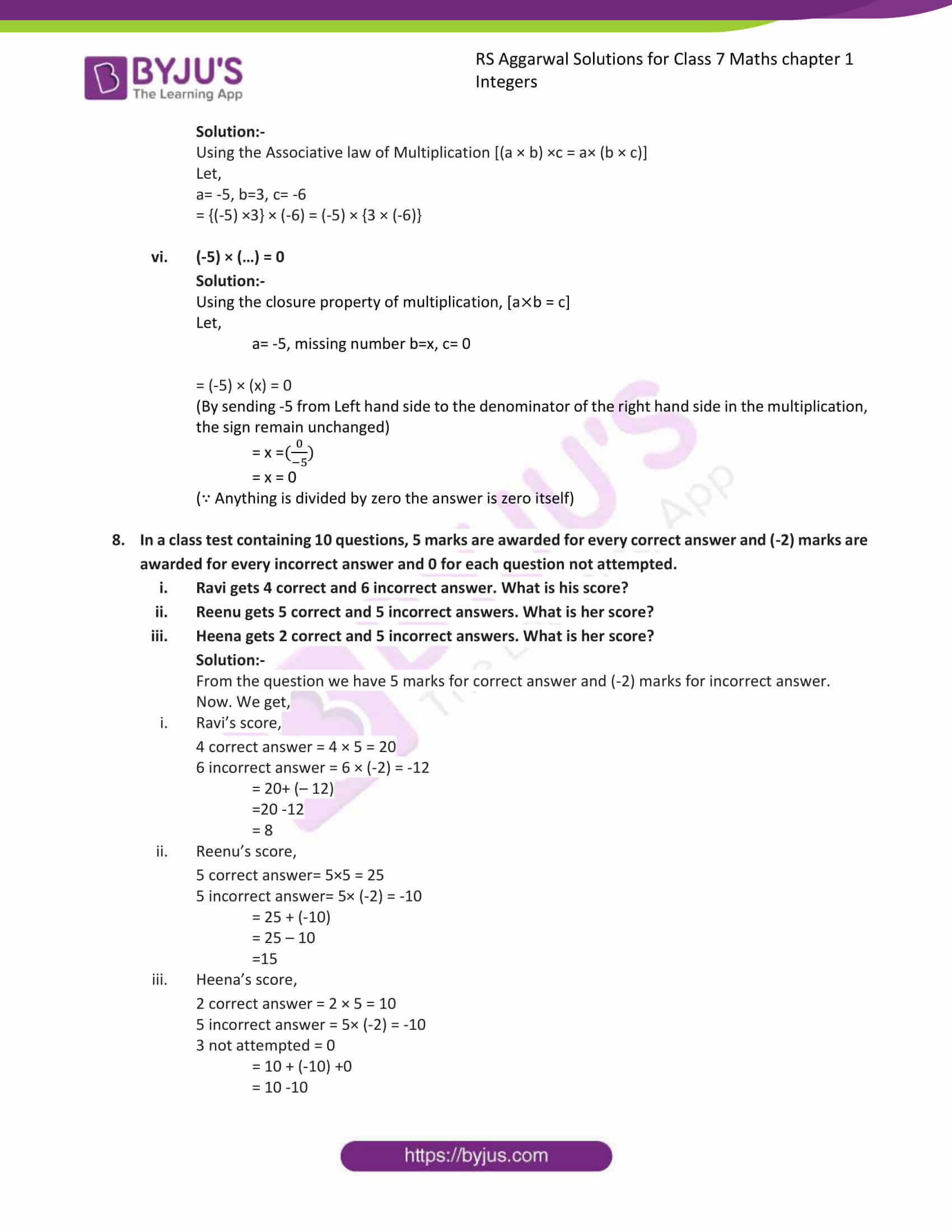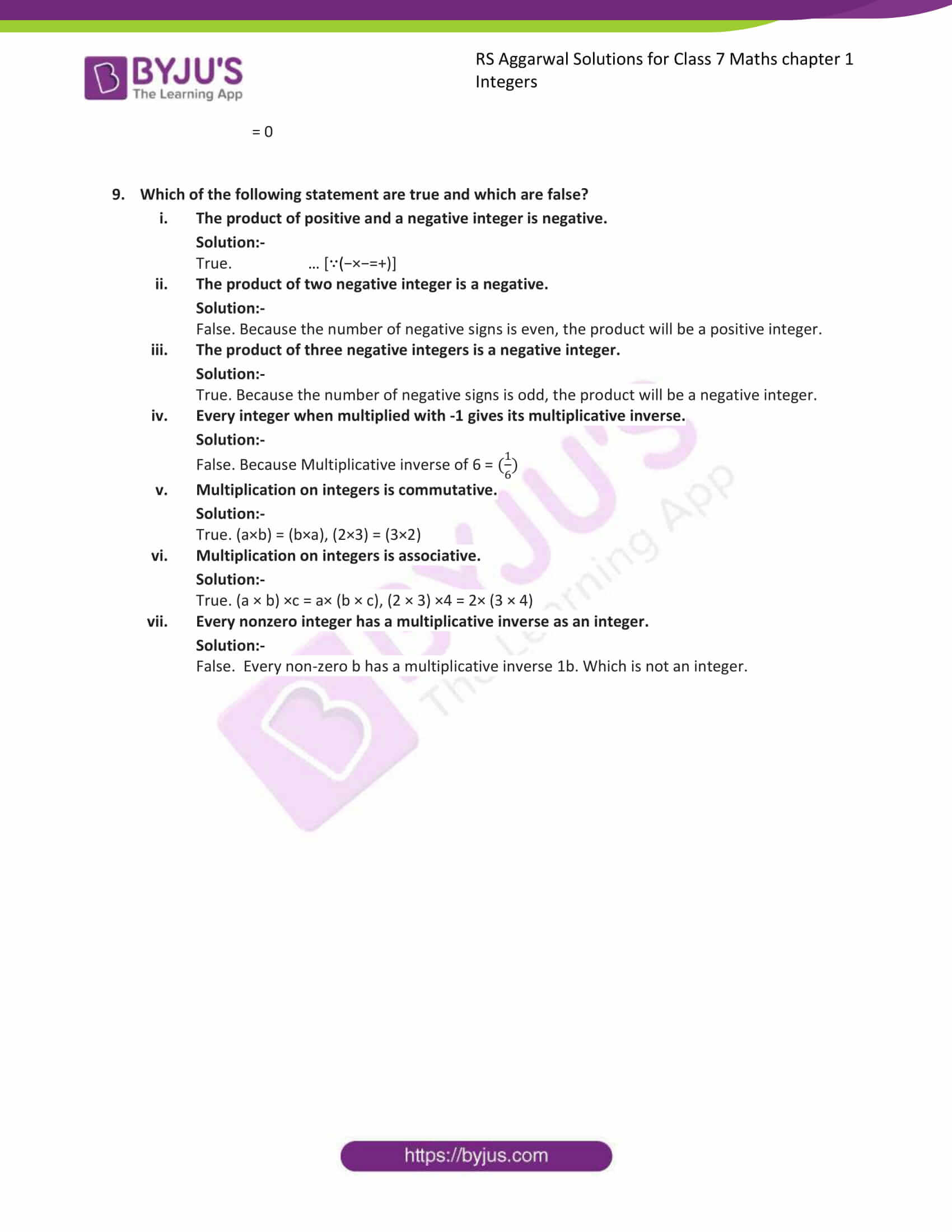### Access answers to Maths RS Aggarwal Solutions for Class 7 Chapter 1 – Integers Exercise 1B

1. Multiply: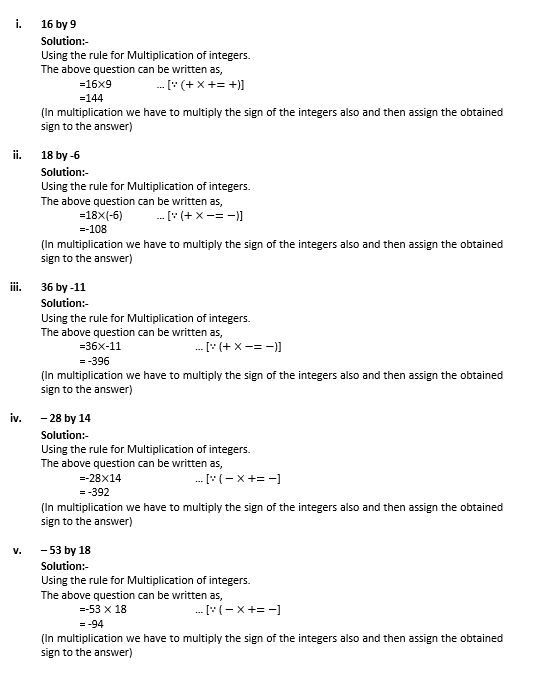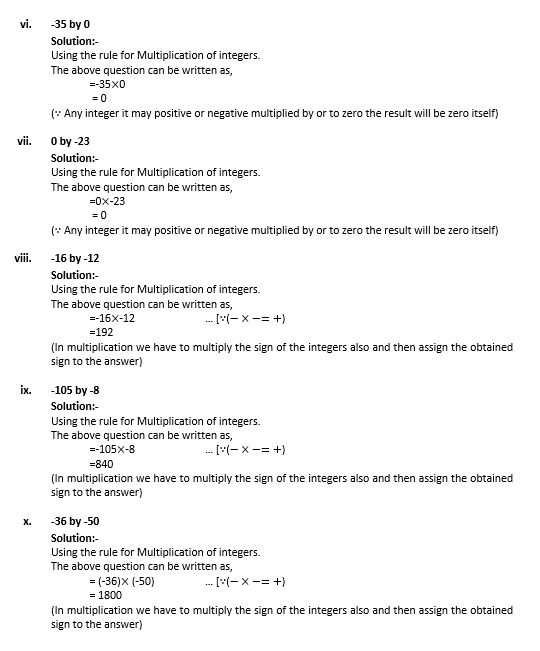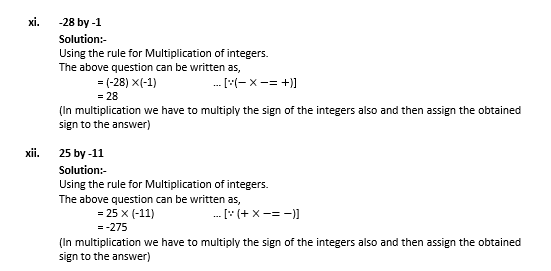1. Find each of the following products: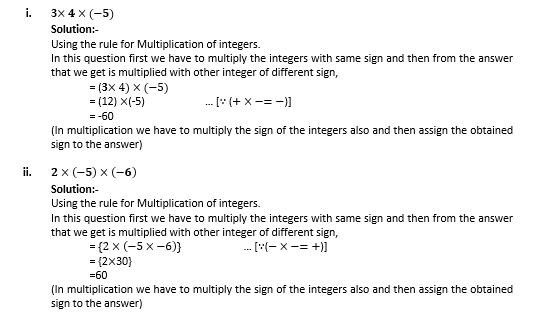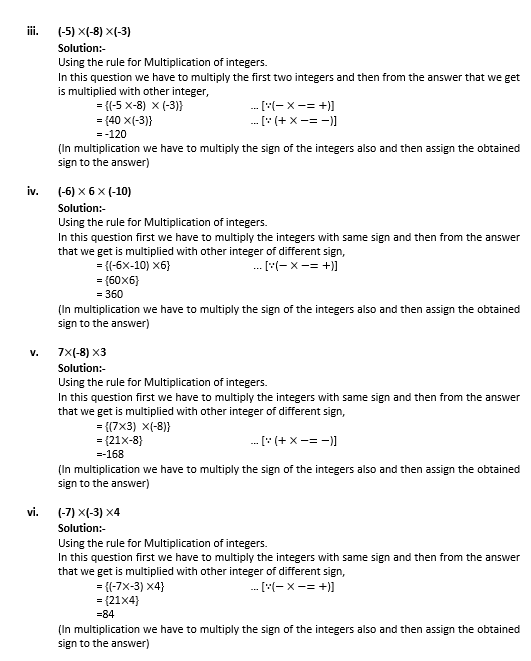2. Find each of the following products: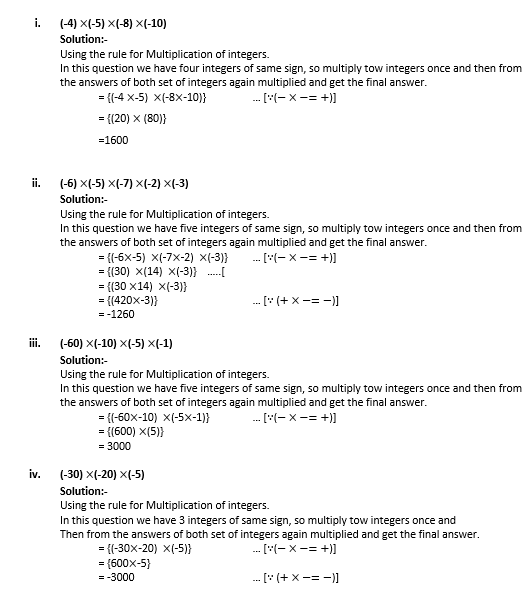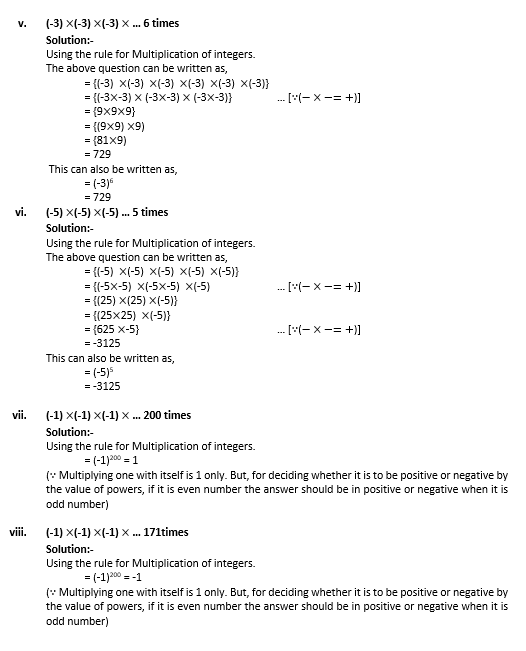4. What will be the sign of the product, if we multiply 90 negative integers and 9 positives integers?

Solution:-

If we multiply the 90 -ve integers the answer we are getting is positive integer. Because, 90 is an even number and the product of even number of negative integer is positive. The product of number of positive integer is not affect the sign of product. So the product obtained from this is positive.

5. What will be the sign of the product, if we multiply 103 negative integers and 65 positives integers?

Solution:-

If we multiply the 103 -ve integers the answer we are getting is negative integer. Because, 103 is an odd number and the product of odd number of negative integer is negative. The product of number of positive integer is not affect the sign of product. So the product obtained from this is negative.

6. Simplify: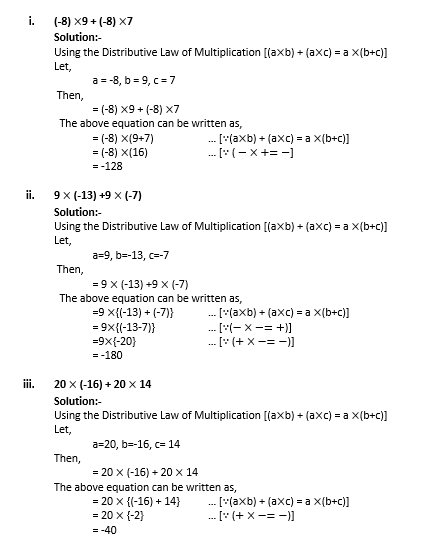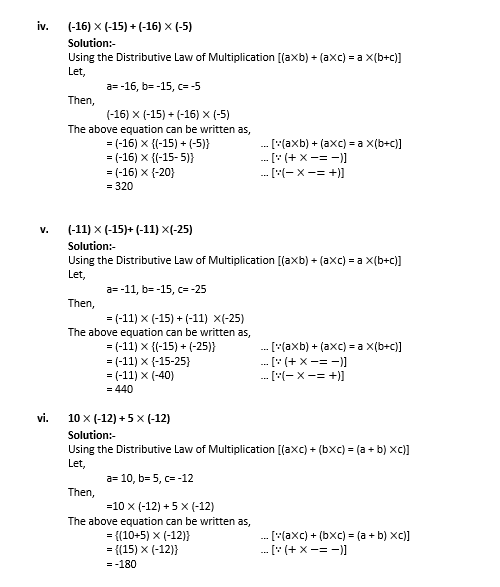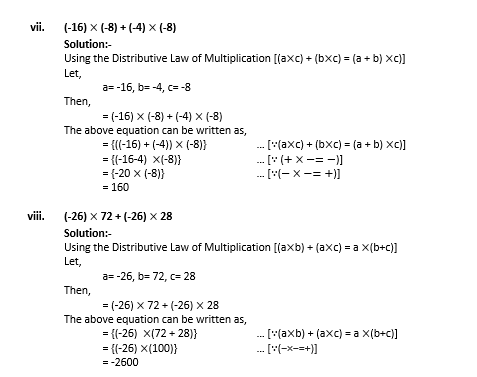7. Fill in the blank: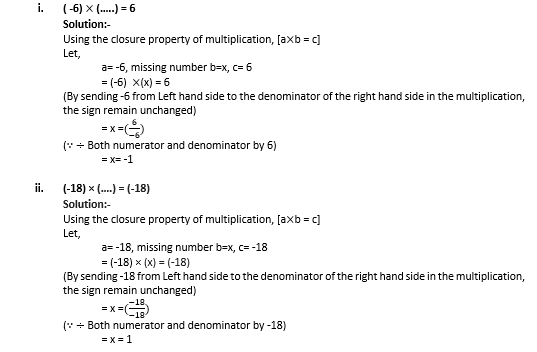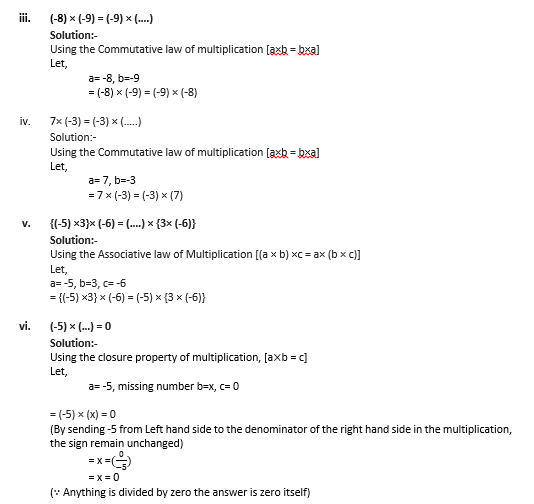8. In a class test containing 10 questions, 5 marks are awarded for every correct answer and (-2) marks are awarded for every incorrect answer and 0 for each question not attempted.
(i) Ravi gets 4 correct and 6 incorrect answer. What is his score?
(ii) Reenu gets 5 correct and 5 incorrect answers. What is her score?
(iii) Heena gets 2 correct and 5 incorrect answers. What is her score?

Solution:-

From the question we have 5 marks for correct answer and (-2) marks for incorrect answer.

Now. We get,

(i) Ravi’s score,

4 correct answer = 4 × 5 = 20

6 incorrect answer = 6 × (-2) = -12

= 20+ (– 12)

= 20 – 12

= 8

(ii) Reenu’s score,

5 correct answer= 5×5 = 25

5 incorrect answer= 5× (-2) = -10

= 25 + (-10)

= 25 – 10

=15

(iii) Heena’s score,

2 correct answer = 2 × 5 = 10

5 incorrect answer = 5× (-2) = -10

3 not attempted = 0

= 10 + (-10) +0

= 10 -10

= 0

9. Which of the following statement are true and which are false?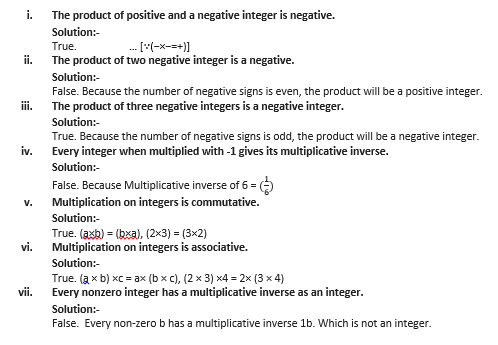### RS Aggarwal Solutions for Class 7 Maths Exercise 1B Chapter – 1 Integers

RS Aggarwal Solutions For Class 7 Maths Chapter 1 Integers Exercise 1B has problems which are based on the Multiplication of Integers and properties of multiplication of integers. This exercise contains some word problems to make the students think in different ways. Students are suggested to try solving the questions from RS Aggarwal Solutions book of Class 7 and then refer to these solutions to know the best way of approaching the different questions.